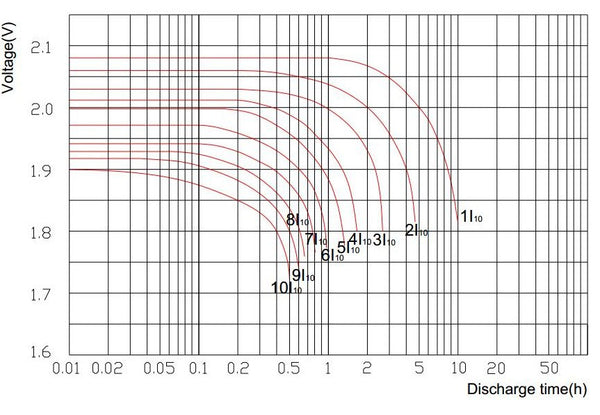The lead-acid battery is put into operation, it is the discharge of the actual load, and its discharge rate depends on the demand of the load. In order to analyze the damage of the battery after long-term use or to estimate the battery's continuous discharge time, its capacity needs to be tested. The following aspects should be considered when investigating the discharge method of battery capacity:

① Depth of discharge, that is, full discharge or partial discharge;

② Discharge rate, that is, discharge at a high discharge rate or a low discharge rate. The discharge methods at various discharge hour rates are as follows. The fully charged battery is allowed to stand for 1~24h, so that the surface temperature of the battery is (25±5)℃, and the electric bicycle battery is discharged to 1.75V/cell (10.5V/pc) with 0.5C2A current, which is called the 2h rate The discharge.

Regardless of their chemical composition, all batteries will self-discharge. The discharge rate of these batteries depends on the operating temperature or the storage. At 80 degrees Fahrenheit, the battery will self-discharges about 4 percent every week. A 125 amp hour rating will self-discharge at about five amps a week. With this in mind, if a 125 Ah battery were stored in the winter for about four months without charging, it would lose 80 amps of its 125 amp capacity. It will have strong sulfation, leading to additional capacity loss. So, preserve your lead-acid batteries charged when not in use.

The ideal discharge curve of a lead acid battery is on a flat discharge curve, the amount of current that the battery can deliver remain more or less constant for quite a while and then drop off rapidly when the limit of it capacity has been reach. The total battery capacity in amp hours x time multiple by the current is ampere hour, is the area under the curve. The bigger and more massive the battery, the greater the area under the curve, the important characteristic is that the will be large enough to operate the equipment it wanted to. It will have a declining discharge curve. The amount of battery current you can get decline more or less right from the start which is not a good thing. Most battery design for cell phones and battery computer, the engineers are taking pains to enjoy you have a flat discharge curve and not a declining discharge curve.

The perfect discharge curve for a lead-acid battery is on a flat discharge curve, the amount of current the battery can deliver remains less constant for a long time and then rapidly decreases when it reached the limit of its capacity. The total battery capacity in amp-hours x time, multiply by the current is the ampere-hours. The bigger and massive the battery, the more area under the curve, the important characteristic is that the battery will be big enough to power the equipment you want. It will have a declining discharge curve. The amount of current from the battery may decline more or less from the beginning and this is not good. For most battery designs for cell phones and battery computers, the engineers strive to ensure you have a flat discharge curve and not a declining discharge curve.

The lead-acid battery discharge curve equation is given by the battery capacity (in ah) divided by the number of hours it takes to discharge the battery. For illustration, a 500 Ah battery capacity that theoretically discharges to a cut-off voltage in 20 hours will have a discharge rate of 500 amps / 20 hours = 25 amps. The battery discharge curve equation allows you to determine the operating time of the battery by finding the inverse slope of the line. This works because the watt-hours divided by the watts give you the runtime hours.

Putting this concept in equation form, you can write E = C x Vavg for the power E in watt-hours, C is the capacity in amp-hours, and Vavg is the average voltage discharge. The Watt-hours offer a suitable way to convert from discharge energy to other forms of energy because multiplying a watt-hour by 3600 to get a watt-hour gives you energy in units of joules.

Learning to look at the discharge curve of lead-acid batteries is an important basis for the selection of lead-acid batteries. It is obvious how long the capacity of a lead-acid battery can be discharged at a certain discharge current, and its termination voltage.

For example, a discharge curves with a capacity of 120AH. If discharge with a current of 120A, the power supply time is about 40 minutes, and the final voltage is about 11.6-11.7V. That is to say, when the battery reaches such a voltage value, it will be very difficult if the battery continues to discharge. It is easy to scrap the battery. It is obvious that the lower the discharge current of the battery, the higher its termination voltage.